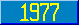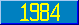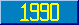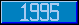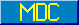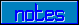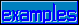## String Relations

### 7.2.2.3 String relations

The relations = ] [ and ]] do not imply any numeric interpretation of either of their operands.

The relation = tests string identity. If the operands are not known to be numeric and numeric equality is to be tested, the programmer may apply an appropriate unary operator to the nonnumeric operands. If both arguments are known to be in numeric form (as would be the case, for example, if they resulted from the application of any operator except _), application of a unary operator is not necessary. The uniqueness of the numeric representation guarantees the equivalence of string and numeric equality when both operands are numeric. Note, however, that the division operator / may produce inexact results, with the usual problems attendant to inexact arithmetic.

The relation [ is called contains. A [ B is true if and only if B is a substring of A; that is, A [ B has the same value as ''\$Find(A,B). Note that the empty string is a substring of every string.

The relation ] is called follows. A ] B is true if and only if A follows B in the sequence, defined here. A follows B if and only if any of the following is true.

1. B is empty and A is not.
2. Neither A nor B is empty, and the leftmost character of A follows (i.e., has a numerically greater \$ASCII value than) the leftmost character of B.
3. There exists a positive integer n such that A and B have identical heads of length n, (i.e., \$Extract(A,1,n) = \$Extract(B,1,n)) and the remainder of A follows the remainder of B (i.e., \$Extract(A,n+1,\$Length(A)) follows \$Extract(B,n+1,\$Length(B))).

The relation ]] is called sorts after. A]]B is true if and only if A follows B in the subscript ordering sequence defined by the single argument \$Order function as if that \$Order refers to a lvn..